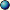### Predefined Parameters : Quaternion

Fractal type Quaternion also has several predefined parameters partially depending on the formula type. They allow you to access some quaternion specific variables like the basis of the three dimensional subspace of the 4 four dimensional quaternion space or the 3D pixel which currently gets examined.

The following parameters exist:Transformation Iteration Coloringalpha real r/w real r/w real r/wBase1 quaternion r/o quaternion r/o quaternion r/oBase2 quaternion r/o quaternion r/o quaternion r/oBase3 quaternion r/o quaternion r/o quaternion r/oBase4 quaternion r/o quaternion r/o quaternion r/oBasepoint quaternion r/o quaternion r/o quaternion r/oe real r/o real r/o real r/oheight real r/o real r/o real r/oindex - – - - – - real r/wmaxiter real r/o real r/o real r/oNormal quaternion r/o quaternion r/o quaternion r/onumiter real r/w real r/w real r/wpi real r/o real r/o real r/opixel complex r/w complex r/w complex r/wpixelcolor - – - - – - color r/wrandom complex r/o complex r/o complex r/orandomrange int r/o int r/o int r/oscreenmax complex r/o complex r/o complex r/oscreenpixel complex r/o complex r/o complex r/osolid bool r/w bool r/w bool r/wwidth real r/o real r/o real r/oz quaternion r/w quaternion r/w quaternion r/wNotes:

The table lists the datatype of the predefined parameter and one of the following abbreviations:

• - – -: not available
• r/o means: read-only
• r/w means: read-write
• w/o means: write-only